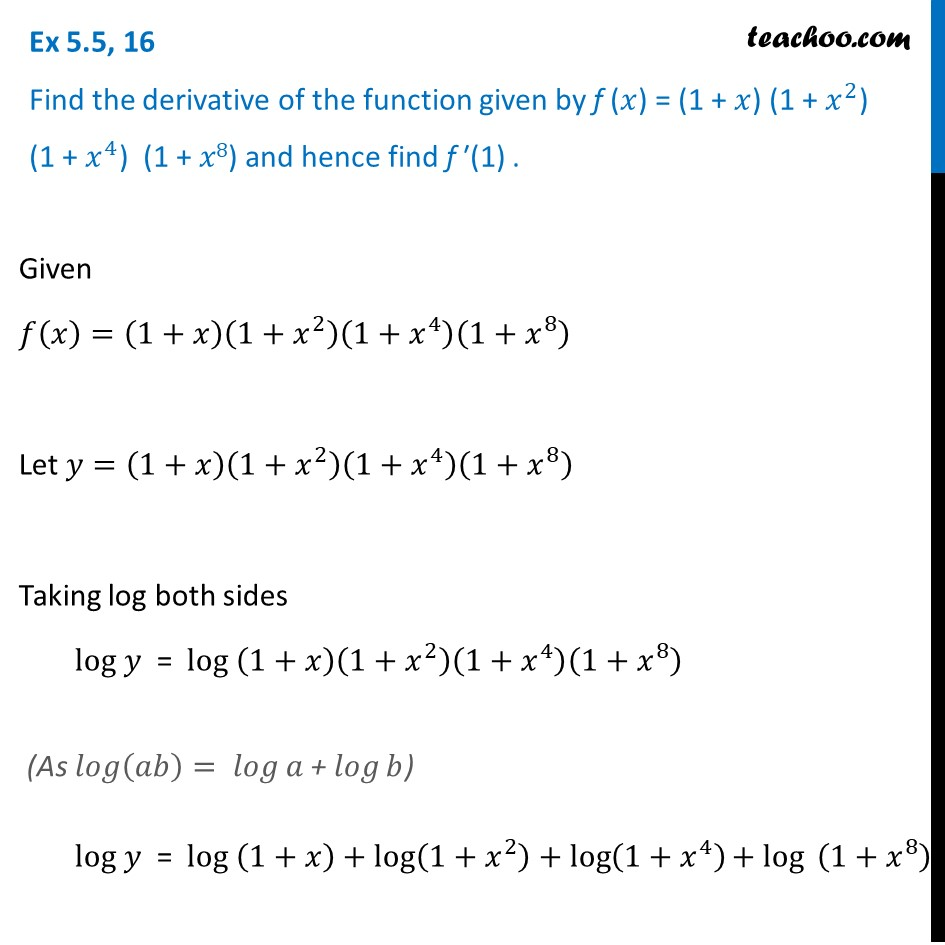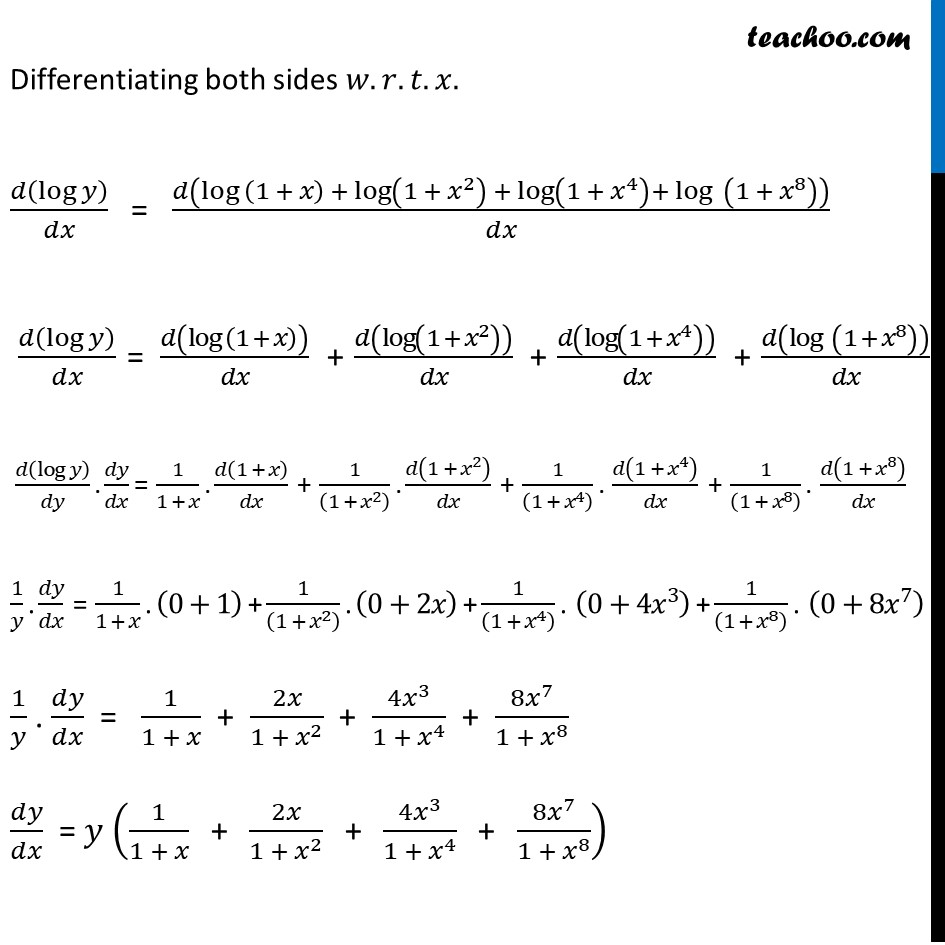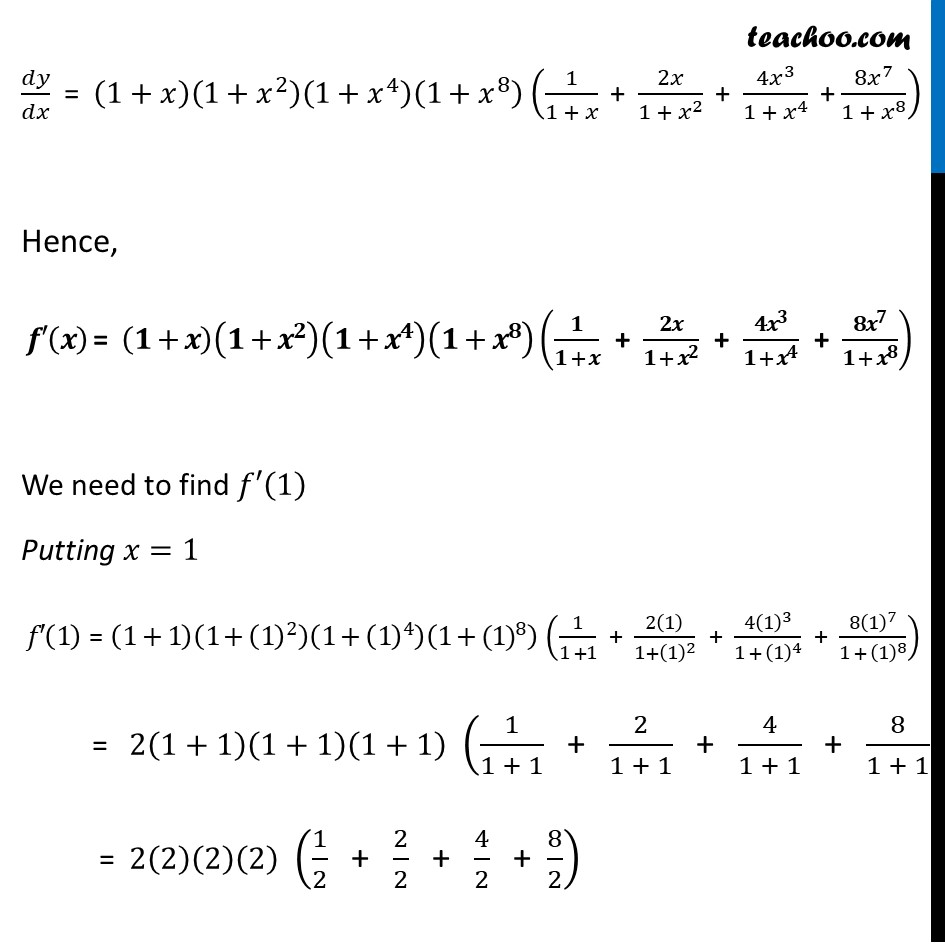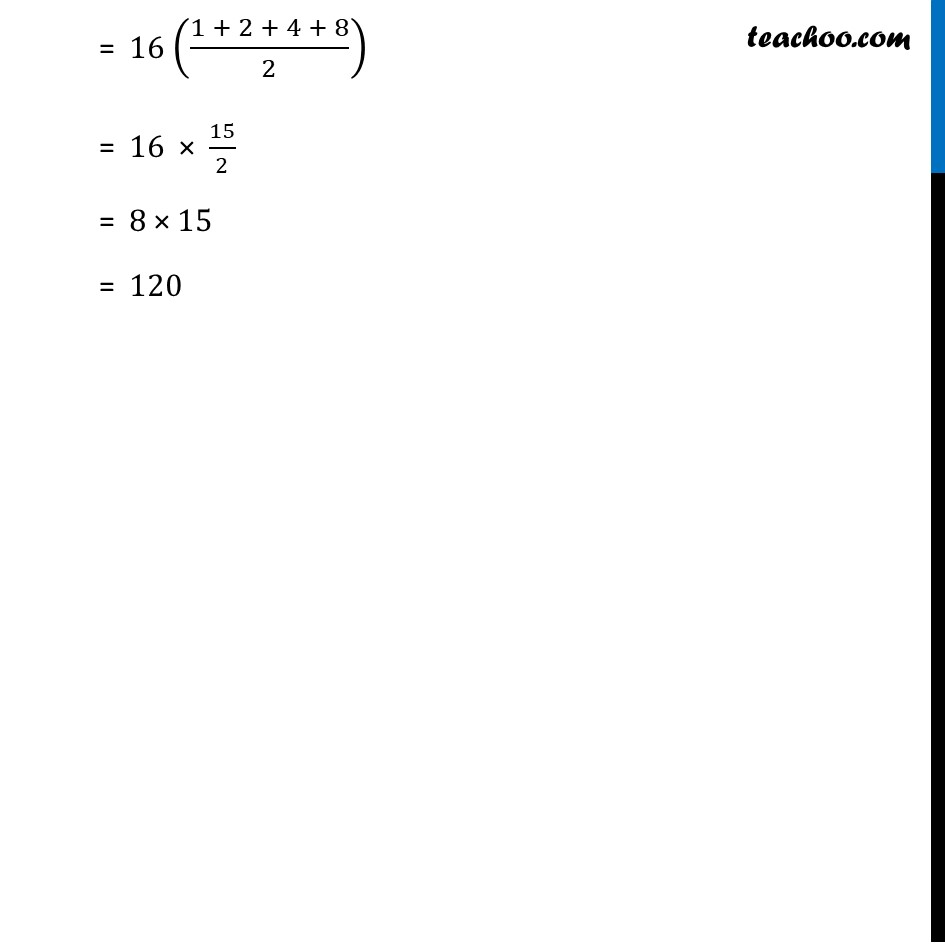Chapter 5 Class 12 Continuity and Differentiability

Class 12
Important Questions for exams Class 12Learn in your speed, with individual attention - Teachoo Maths 1-on-1 Class

### Transcript

Ex 5.5, 16 Find the derivative of the function given by f (𝑥) = (1 + 𝑥) (1 + 𝑥^2) (1 + 𝑥^4) (1 + 𝑥8) and hence find f ′(1) .Given 𝑓(𝑥)=(1+𝑥)(1+𝑥^2 )(1+𝑥^4 )(1+𝑥^8 )" " Let 𝑦=(1+𝑥)(1+𝑥^2 )(1+𝑥^4 )(1+𝑥^8 ) Taking log both sides log 𝑦 = log (1+𝑥)(1+𝑥^2 )(1+𝑥^4 )(1+𝑥^8 ) log 𝑦 = log (1+𝑥)+log⁡(1+𝑥^2 )+log⁡(1+𝑥^4 ) 〖+ log〗⁡〖 (1+𝑥^8 )〗 Differentiating both sides 𝑤.𝑟.𝑡.𝑥. 𝑑(log⁡𝑦 )/𝑑𝑥 = 𝑑(log (1 + 𝑥) + log⁡(1 + 𝑥^2 ) + log⁡(1 + 𝑥^4 )+ log⁡〖 (1 + 𝑥^8 )〗 )/𝑑𝑥 𝑑(log⁡𝑦 )/𝑑𝑥 = 𝑑(log (1 + 𝑥))/𝑑𝑥 + 𝑑(log⁡(1 + 𝑥^2 ) )/𝑑𝑥 + 𝑑(log⁡(1 + 𝑥^4 ) )/𝑑𝑥 + 𝑑(log⁡〖 (1 + 𝑥^8 )〗 )/𝑑𝑥 𝑑(log⁡𝑦 )/𝑑𝑦 . 𝑑𝑦/𝑑𝑥 = 1/(1 + 𝑥) . 𝑑(1 + 𝑥)/𝑑𝑥 + 1/((1 + 𝑥^2 ) ) . 𝑑(1 + 𝑥^2 )/𝑑𝑥 + 1/((1 + 𝑥^4 ) ) . 𝑑(1 + 𝑥^4 )/𝑑𝑥 + 1/((1 + 𝑥^8 ) ) . 𝑑(1 + 𝑥^8 )/𝑑𝑥 1/𝑦 . 𝑑𝑦/𝑑𝑥 = 1/(1 + 𝑥) . (0+1) + 1/((1 + 𝑥^2 ) ) . (0+2𝑥) + 1/((1 + 𝑥^4 ) ) . (0+4𝑥^3 ) + 1/((1 + 𝑥^8 ) ) . (0+8𝑥^7 ) 1/𝑦 . 𝑑𝑦/𝑑𝑥 = 1/(1 + 𝑥) + 2𝑥/(1 + 𝑥^2 ) + (4𝑥^3)/(1 + 𝑥^4 ) + (8𝑥^7)/(1 + 𝑥^8 ) 𝑑𝑦/𝑑𝑥 = 𝑦 (1/(1 + 𝑥) " + " 2𝑥/(1 + 𝑥^2 ) " + " (4𝑥^3)/(1 + 𝑥^4 ) " + " (8𝑥^7)/(1 + 𝑥^8 )) 𝑑𝑦/𝑑𝑥 = (1+𝑥)(1+𝑥^2 )(1+𝑥^4 )(1+𝑥^8 ) (1/(1 + 𝑥) " + " 2𝑥/(1 + 𝑥^2 ) " + " (4𝑥^3)/(1 + 𝑥^4 ) " +" (8𝑥^7)/(1 + 𝑥^8 )) Hence, 𝒇′(𝒙) = (𝟏+𝒙)(𝟏+𝒙^𝟐 )(𝟏+𝒙^𝟒 )(𝟏+𝒙^𝟖 ) (𝟏/(𝟏 + 𝒙) " + " 𝟐𝒙/(𝟏 + 𝒙^𝟐 ) " + " (𝟒𝒙^𝟑)/(𝟏 + 𝒙^𝟒 ) " + " (𝟖𝒙^𝟕)/(𝟏 + 𝒙^𝟖 )) We need to find 𝑓′(1) Putting 𝑥=1 𝑓′(1) = (1+1)(1+(1)^2 )(1+(1)^4 )(1+〖(1)〗^8 ) (1/(1 +1) " + " 2(1)/(1+(1)^2 ) " + " (4(1)^3)/(1 + (1)^4 ) " + " (8(1)^7)/(1 + (1)^8 )) = 2(1+1)(1+1)(1+1) (1/(1 + 1) " + " 2/(1 + 1) " + " 4/(1 + 1) " + " 8/(1 + 1)) = 2(2)(2)(2) (1/2 " + " 2/2 " + " 4/2 " + " 8/2) 𝑑𝑦/𝑑𝑥 = (1+𝑥)(1+𝑥^2 )(1+𝑥^4 )(1+𝑥^8 ) (1/(1 + 𝑥) " + " 2𝑥/(1 + 𝑥^2 ) " + " (4𝑥^3)/(1 + 𝑥^4 ) " +" (8𝑥^7)/(1 + 𝑥^8 )) Hence, 𝒇′(𝒙) = (𝟏+𝒙)(𝟏+𝒙^𝟐 )(𝟏+𝒙^𝟒 )(𝟏+𝒙^𝟖 ) (𝟏/(𝟏 + 𝒙) " + " 𝟐𝒙/(𝟏 + 𝒙^𝟐 ) " + " (𝟒𝒙^𝟑)/(𝟏 + 𝒙^𝟒 ) " + " (𝟖𝒙^𝟕)/(𝟏 + 𝒙^𝟖 )) We need to find 𝑓′(1) Putting 𝑥=1 𝑓′(1) = (1+1)(1+(1)^2 )(1+(1)^4 )(1+〖(1)〗^8 ) (1/(1 +1) " + " 2(1)/(1+(1)^2 ) " + " (4(1)^3)/(1 + (1)^4 ) " + " (8(1)^7)/(1 + (1)^8 )) = 2(1+1)(1+1)(1+1) (1/(1 + 1) " + " 2/(1 + 1) " + " 4/(1 + 1) " + " 8/(1 + 1)) = 2(2)(2)(2) (1/2 " + " 2/2 " + " 4/2 " + " 8/2)